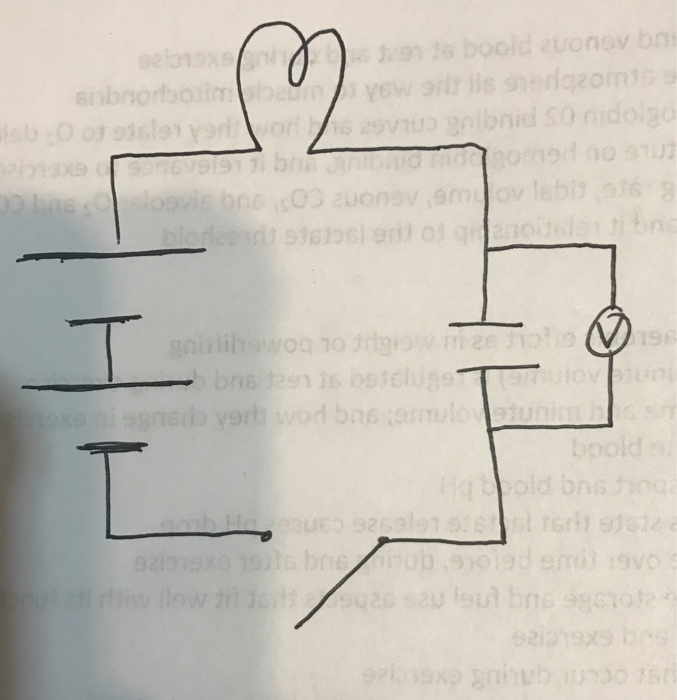# Problem: Describe qualitatively but specifically how the circuit quantities change after the switch is closed in the circuit a) current b) voltage across the capicitor. Explain why these quantities change as they do, in terms of motion and presence of the capacitor.

###### FREE Expert Solution

a)

If the switch is closed, the capacitor becomes a short circuit.

The current in this circuit is given by:

$\overline{){\mathbf{i}}{\mathbf{=}}\frac{{\mathbf{V}}_{\mathbf{1}}\mathbf{+}{\mathbf{V}}_{\mathbf{2}}}{\mathbf{R}}}$

93% (211 ratings)###### Problem Details

Describe qualitatively but specifically how the circuit quantities change after the switch is closed in the circuit a) current b) voltage across the capicitor. Explain why these quantities change as they do, in terms of motion and presence of the capacitor.